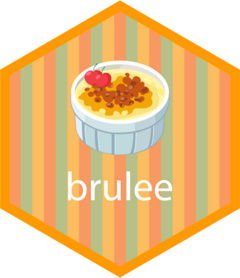# bruleeThe R `brulee` package contains several basic modeling functions that use the `torch` package infrastructure, such as:

## Installation

You can install the released version of brulee from CRAN with:

``install.packages("brulee")``

And the development version from GitHub with:

``````# install.packages("devtools")
devtools::install_github("tidymodels/brulee")``````

## Example

`brulee` has formula, x/y, and recipe user interfaces for each function. For example:

``````library(brulee)
library(recipes)
library(yardstick)

data(bivariate, package = "modeldata")
set.seed(20)
nn_log_biv <- brulee_mlp(Class ~ log(A) + log(B), data = bivariate_train,
epochs = 150, hidden_units = 3)

# We use the tidymodels semantics to always return a tibble when predicting
predict(nn_log_biv, bivariate_test, type = "prob") %>%
bind_cols(bivariate_test) %>%
roc_auc(Class, .pred_One)
#> # A tibble: 1 × 3
#>   .metric .estimator .estimate
#>   <chr>   <chr>          <dbl>
#> 1 roc_auc binary         0.410``````

A recipe can also be used if the data require some sort of preprocessing (e.g., indicator variables, transformations, or standardization):

``````library(recipes)

rec <-
recipe(Class ~ ., data = bivariate_train) %>%
step_YeoJohnson(all_numeric_predictors()) %>%
step_normalize(all_numeric_predictors())

set.seed(20)
nn_rec_biv <- brulee_mlp(rec, data = bivariate_train,
epochs = 150, hidden_units = 3)

# A little better
predict(nn_rec_biv, bivariate_test, type = "prob") %>%
bind_cols(bivariate_test) %>%
roc_auc(Class, .pred_One)
#> # A tibble: 1 × 3
#>   .metric .estimator .estimate
#>   <chr>   <chr>          <dbl>
#> 1 roc_auc binary         0.708``````

## Code of Conduct

Please note that the brulee project is released with a Contributor Code of Conduct. By contributing to this project, you agree to abide by its terms.